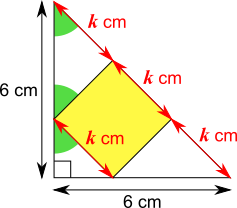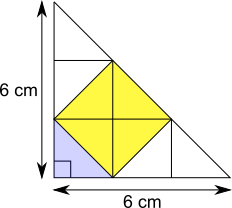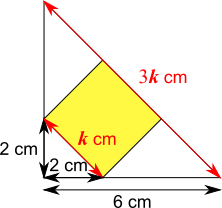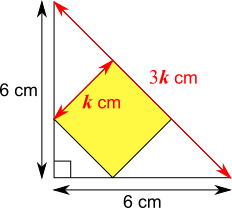#### You may also like### Some(?) of the Parts

A circle touches the lines OA, OB and AB where OA and OB are perpendicular. Show that the diameter of the circle is equal to the perimeter of the triangle### Ladder and Cube

A 1 metre cube has one face on the ground and one face against a wall. A 4 metre ladder leans against the wall and just touches the cube. How high is the top of the ladder above the ground?### Bendy Quad

Four rods are hinged at their ends to form a convex quadrilateral. Investigate the different shapes that the quadrilateral can take. Be patient this problem may be slow to load.

# Square and Triangle

##### Age 14 to 16 ShortChallenge LevelThe sides of the large right-angled triangle are both 6 cm, so its angles are 90$^\circ$, 45$^\circ$, and 45$^\circ$.

The angles in the square are all right angles, so the smaller triangles around the square are also right-angled isosceles triangles.

This means that the angles shaded green on the diagram are all 45$^\circ$, and the lengths labelled $k$ cm are all equal to the side length of the square.

This is important for all of the three methods shown below:

Using congruent trianglesSince the lines in the diagram are all horizontal, vertical or at $45^\circ$, it can be split up using horizontal and vertical lines as shown. Now all of the triangles are congruent to the smallest triangle, at the bottom left corner of the original diagram.

There are 9 of these triangles altogether, and 4 of them fit into the square, so the area of the square is $\frac49$ of the area of the whole triangle.

The area of the whole triangle is $\frac12\times6\times6 = 18$ cm$^2$. So the area of the square is $\frac49$ of $18$ cm$^2$, which is $8$ cm$^2$.

Using scale factors
The diagonal side of the whole triangle is equal to 3$k$ cm, and the diagonal side of the small triangle in its corner (to the bottom left of the square) is $k$ cm. So the scale factor from the small triangle to the whole triangle is 3.This means that the sides of the small triangle are 2 cm, since 2 cm $\times$ 3 = 6 cm.

So the area of the small triangle is 2$\times$2$\div$2 = 2 cm$^2$.

The area of the whole triangle is 6$\times$6$\div$2 = 18 cm$^2$.

So the area of the square and the two triangles on either side of it is 18 $-$ 2 = 16 cm$^2$. Each of these two triangles is congruent to half of the square, so the square occupies half of this area. So the area of the square is 8 cm$^2$.

Using Pythagoras' Theorem on the whole triangleThe hypotenuse of the triangle is equal to $3k$, and can also be found using Pythagoras' Theorem: $$6^2+6^2=(3k)^2\\\Rightarrow 72=9k^2\\ \Rightarrow 8=k^2$$ But $k^2$ is the area of the square. So the area of the square is $8$ cm$^2$.

You can find more short problems, arranged by curriculum topic, in our short problems collection.